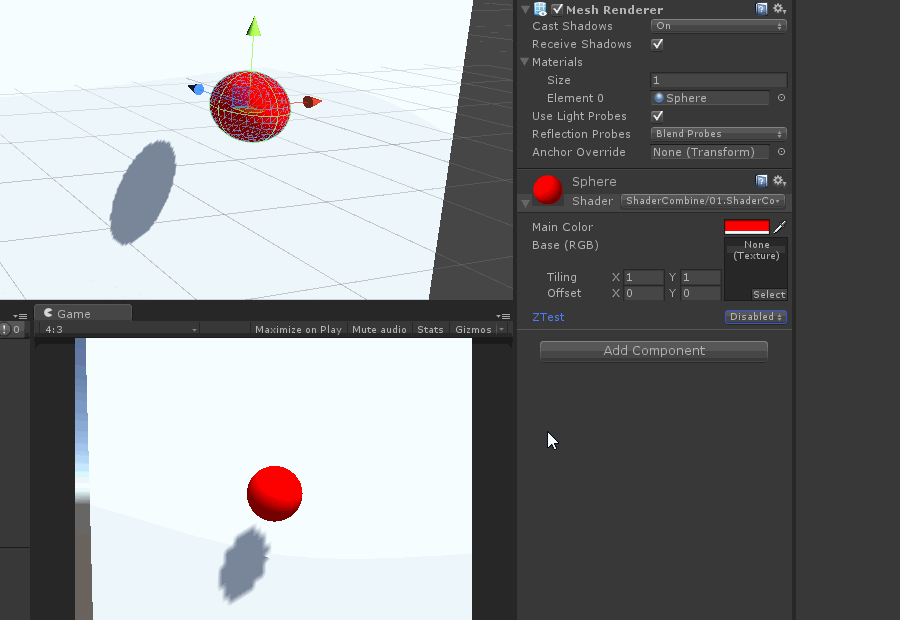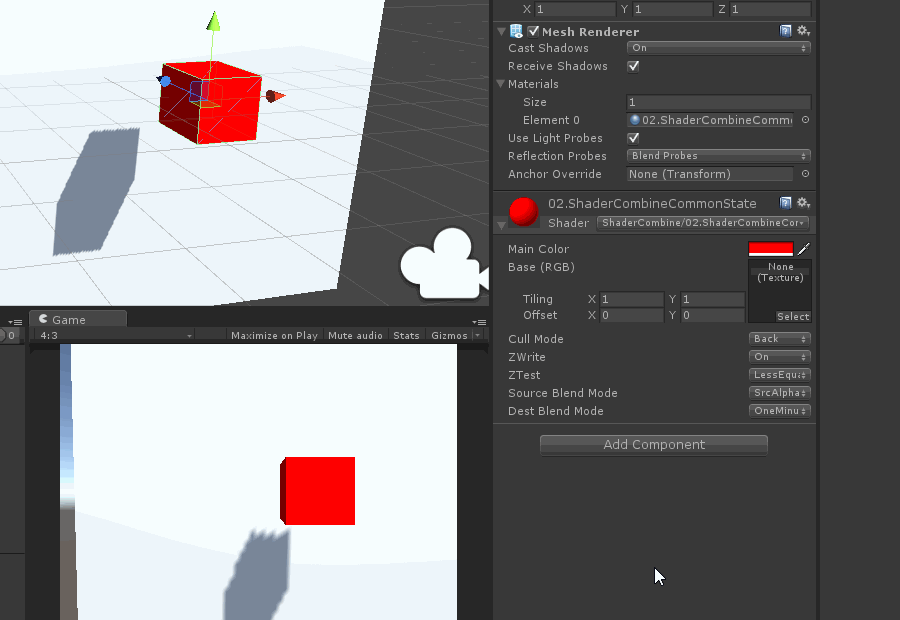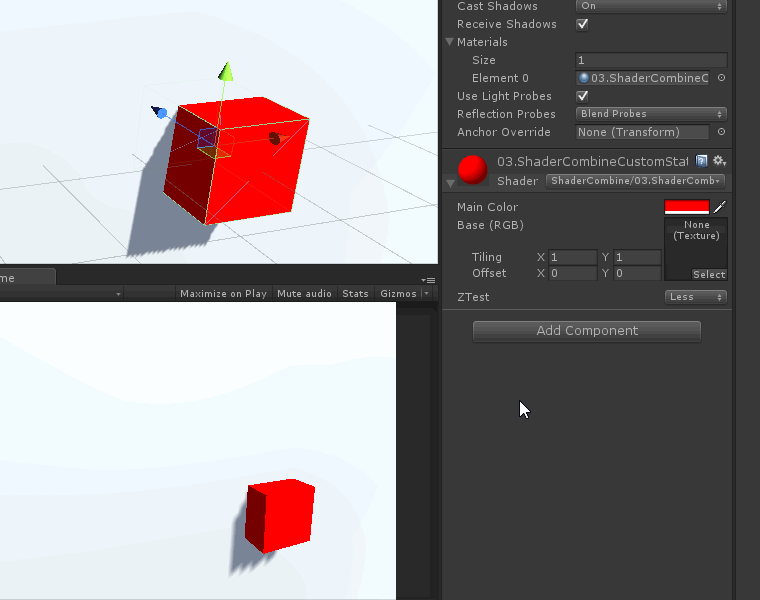◆◆

``````Normal-Diffuse.shader(Legacy Shaders/Diffuse)
``````

1.2 在属性列表(Properties)中添加一行

``````[Enum(UnityEngine.Rendering.CompareFunction)] _ZTest ("ZTest", Float) = 2
``````

``````ZTest [_ZTest]
``````

``````Shader "ShaderCombine/01.ShaderCombineSimpleZTest"
{
Properties {
_Color ("Main Color", Color) = (1,1,1,1)
_MainTex ("Base (RGB)", 2D) = "white" {}
[Enum(UnityEngine.Rendering.CompareFunction)] _ZTest ("ZTest", Float) = 2
}
Tags { "RenderType"="Opaque" }
LOD 200
ZTest [_ZTest]

CGPROGRAM
#pragma surface surf Lambert

sampler2D _MainTex;
fixed4 _Color;

struct Input {
float2 uv_MainTex;
};

void surf (Input IN, inout SurfaceOutput o) {
fixed4 c = tex2D(_MainTex, IN.uv_MainTex) * _Color;
o.Albedo = c.rgb;
o.Alpha = c.a;
}
ENDCG
}

}
``````

1.5 直观展示◆◆

``````Shader "ShaderCombine/02.ShaderCombineCommonState"
{
Properties {
_Color ("Main Color", Color) = (1,1,1,1)
_MainTex ("Base (RGB)", 2D) = "white" {}
[Enum(UnityEngine.Rendering.CullMode)] _Cull ("Cull Mode", Float) = 1
[Enum(Off,0,On,1)] _ZWrite ("ZWrite", Float) = 1
[Enum(UnityEngine.Rendering.CompareFunction)] _ZTest ("ZTest", Float) = 1
[Enum(UnityEngine.Rendering.BlendMode)] _SourceBlend ("Source Blend Mode", Float) = 2
[Enum(UnityEngine.Rendering.BlendMode)] _DestBlend ("Dest Blend Mode", Float) = 2
}
Tags { "RenderType"="Opaque" }
LOD 200

ZTest [_ZTest]
Cull [_Cull]
ZWrite [_ZWrite]
ZTest [_ZTest]
Blend [_SourceBlend] [_DestBlend]

CGPROGRAM
#pragma surface surf Lambert

sampler2D _MainTex;
fixed4 _Color;

struct Input {
float2 uv_MainTex;
};

void surf (Input IN, inout SurfaceOutput o) {
fixed4 c = tex2D(_MainTex, IN.uv_MainTex) * _Color;
o.Albedo = c.rgb;
o.Alpha = c.a;
}
ENDCG
}

}
``````

2.2 直观展示◆◆◆

3.1 剔除模式

``````UnityEngine.Rendering.CullMode：
public enum CullMode
{
Off   = 0,    //Disable culling.
Front = 1,    //Cull front-facing geometry.
Back  = 2     //Cull back-facing geometry.
}
``````

3.2 比较方式

``````
UnityEngine.Rendering.CompareFunction
//Depth or stencil comparison function.
public enum CompareFunction
{
Disabled     = 0,   //Depth or stencil test is disabled.
Never        = 1,   //Never pass depth or stencil test.
Less         = 2,   //Pass depth or stencil test when new value is less than old one.
Equal        = 3,   //Pass depth or stencil test when values are equal.
LessEqual    = 4,   //Pass depth or stencil test when new value is less or equal than old one.
Greater      = 5,   //Pass depth or stencil test when new value is greater than old one.
NotEqual     = 6,   //Pass depth or stencil test when values are different.
GreaterEqual = 7,   //Pass depth or stencil test when new value is greater or equal than old one.
Always       = 8    //Always pass depth or stencil test.
}
``````

3.3 混合模式

``````UnityEngine.Rendering.BlendMode
//Blend mode for controlling the blending.
public enum BlendMode
{
Zero             = 0,   //Blend factor is (0, 0, 0, 0).
One              = 1,   //Blend factor is (1, 1, 1, 1).
DstColor         = 2,   //Blend factor is (Rd, Gd, Bd, Ad).
SrcColor         = 3,   //Blend factor is (Rs, Gs, Bs, As).
OneMinusDstColor = 4,   //Blend factor is (1 - Rd, 1 - Gd, 1 - Bd, 1 - Ad).
SrcAlpha         = 5,   //Blend factor is (As, As, As, As).
OneMinusSrcColor = 6,   //Blend factor is (1 - Rs, 1 - Gs, 1 - Bs, 1 - As).
SrcAlphaSaturate = 9,   //Blend factor is (f, f, f, 1); where f = min(As, 1 - Ad).
OneMinusSrcAlpha = 10   //Blend factor is (1 - As, 1 - As, 1 - As, 1 - As).
}
``````

3.4 有限的自定义

``````[Enum(UnityEngine.Rendering.CompareFunction)] _ZTest ("ZTest", Float) = 2
``````

``````[Enum(Less,2,Greater,5)] _ZTest ("ZTest", Float) = 2
``````

``````Shader "ShaderCombine/03.ShaderCombineCustomState"
{
Properties {
_Color ("Main Color", Color) = (1,1,1,1)
_MainTex ("Base (RGB)", 2D) = "white" {}
[Enum(Less,2,Greater,5)] _ZTest ("ZTest", Float) = 2
}
Tags { "RenderType"="Opaque"}
LOD 200
ZTest [_ZTest]

CGPROGRAM
#pragma surface surf Lambert

sampler2D _MainTex;
fixed4 _Color;

struct Input {
float2 uv_MainTex;
};

void surf (Input IN, inout SurfaceOutput o) {
fixed4 c = tex2D(_MainTex, IN.uv_MainTex) * _Color;
o.Albedo = c.rgb;
o.Alpha = c.a;
}
ENDCG
}

}

``````

3.5 直观展示PS：其实最初是想花一整章篇幅来讲解MaterialPropertyDrawer的各种使用，但其内部的扩展空间还比较广泛。写下来篇幅太长，而太长的篇幅，阅读起来也比较麻烦，所以还是拆成几章篇幅来慢慢絮叨吧，同时也遵从一次只讲一个问题。